ABACUS: MYSTERY OF THE BEAD The Bead Unbaffled - An Abacus Manual

###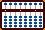Kato Fukutaro's Square Roots(contributed by Edvaldo Siqueira)

The following is a method used by Professor Fukutaro Kato, a Japanese soroban teacher living in Brazil in the 1960's. The method was published in the Professor's book, *SOROBAN pelo Método Moderno* which, when translated from Portuguese means *SOROBAN by the Modern Method.

I will try to expose Kato's ideas for extracting the *square root*. If I remember well, Kojima mentions *en passant* this method. Before the method itself, it is useful to grasp the three following concepts.

I) ONE HALF OF A SQUARED NUMBER (Although one gains speed in learning by heart the table below, its memorization is not strictly necessary while extracting the square root.)

 `Number Its Square Half its Square` ```1 --------- 1 --------- 0.5 2 --------- 4 --------- 2.0 3 --------- 9 --------- 4.5 4 --------- 16 --------- 8.0 5 --------- 25 --------- 12.5 6 --------- 36 --------- 18.0 7 --------- 49 --------- 24.5 8 --------- 64 --------- 32.0 9 --------- 81 --------- 40.5 ```

II) HOW TO HALF A NUMBER ON THE SOROBAN (WITHOUT DISPLACING THE ORDER)

Here we don't want to displace the order of the numbers on the soroban. Multiplying by 0.5 is the same as dividing by 2  (for some it may be easier to multiply than to divide.  For the best results use the modern multiplication technique.) As an example lets say we had the number 325, as below on D,E & F. Multiplying 325 x 0.5 = 1625.

 ```A B C D E F G H 0 0 0 3 2 5 0 0 ``` ```A B C D E F G H 0 0 0 1 6 2 5 0 ```

As an alternative one could think of it this way. Instead of multiplying by 0.5 subtract one half of each number. Starting on the right hand side and working left take away ½ of  5 on F leaving 2.5 on FG. Take away ½  of 2 on E leaving it 1. Finally take away ½ of 3 on D leaving 1.5 on D & E. This yeilds the desired 1625 on rods DEFG.

III) PLACING NUMBERS ON THE SOROBAN

With regards to setting numbers on the soroban, Professor Fukutaro explains it this way;

a) The radicand has to be set so that the its unit falls always on a
unit rod.

b) When a number is not a perfect square, one must pay attention
that the remainder unit should always fall also on that unit rod.

c) To set the square root (the result), one must follow these
principles:

ci) If the radicand is a complete 2-digit group number,
(like 1225), set its square root highest digit (3) exactly to the

cii) If the radicand is an incomplete 2-digit group number
(like 15129), skip one column to the left to set the square root
highest order digit (1 in this case).

With these notions (I, II  & III) in mind, lets try to find the square root of *1225*

 Before continuing, it may be helpful to visit these links; ===> Gary Flom contributed this algebraic explanation of how the method works: Gary Flom's Explanation ===> Professor Fukutaro's algebraic & graphic explanation : Professor Fukutaro's Explanations ===> Further algebraic & graphic explanations by Professor Fukutaro's : Portuguese Explanation ===> For a more extensive explanation on number placement: Number Placement

Example 1: \/ 1225  = 35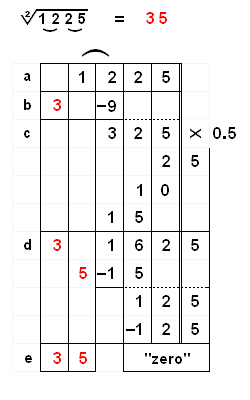a) Mentally separate the the number 1225 into groups of 2 numbers each, 12  25. b) Look at the first group (12). Find the largest perfect square that is less than or equal to 12. The answer is 3. (Square this 3 = 9). Subtract 9 from 12. We're left with 325. c) Take half of 325 (multiply by 0.5). The result is 162.5 d) To find the second digit, divide 3 into 16. The largest integer is 5. d) Multiply the 1st calculated digit by the 2nd. (3 x 5) and subtract the product 15 from 16. We are left with 12.5. e) Subtract *half* the square of 5 = 12.5 (see the above table, if you have not already learned it by heart.) We are left with "zero" That's it! We have just found the square root. It must be 35.

Example 2: \/ 362404  = 602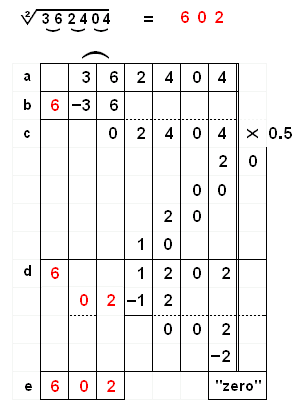a) Mentally separate the the number 362404 into groups of 2 numbers each, 36  24  04. b) Look at the first group (36). Find the largest perfect square that is less than or equal to 36. The answer is 6. (Square this 6 =36). Subtract 36 from the first group, leaving 02404. c) Take half of 2404 (multiply by 0.5). The result is 1202 d) To find the second digit, divide 6 into 1. It can't be done so move on to divide 6 into 12. The largest integer is 2. d) Multiply the 1st calculated digit by the 2nd. (6 x 2) and subtract the product 12 from 12. We are left with 2. e) Subtract *half* the square of 2 = 2 (see the above table). We are left with "zero" The answer to the problem is 602.

Example 3: \/ 0.2025  = 0.45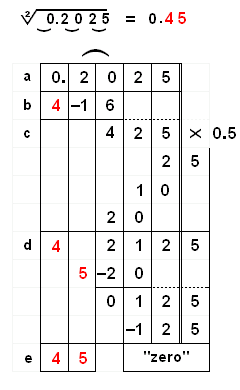a) Mentally separate the the number 0.2025 into groups of 2 numbers each, 0   20  25. b) Here the first workable group is 20. It's largest perfect square is 4. (Square this 4 =16). Subtract 16 from the first group, leaving 425. c) Take half of 425 (multiply by 0.5). The result is 212.5 d) To find the second digit, divide 4 into 21. The largest integer is 5. d) Multiply the 1st calculated digit by the 2nd. (4 x 5) and subtract the product 20 from 21. We are left with 2. e) Subtract *half* the square of 5 = 12.5 (see the above table). We are left with "zero" The answer to the problem is 0.45

Example 4: \/ 228520  = 478

*** This is an interesting example because it shows how easy it is to revise an answer in the event of a mistake.***a) Mentally separate the the number 228520 into groups of 2 numbers each, 22   85  20. b) We know the first number in the answer will be 4. (Square this 4 =16). Subtract 16 from the first group 22, leaving 68520. c) Take half of 68520 (multiply by 0.5). The result l is 34260 d) To find the second digit, divide 4 into 34. The largest integer is 8. Multiply 4 x 8 and subtract the product 32 from 34. Now the method requires subtracting *half* the square. (8 *8) = 64 /2 = 32. But here's the problem. *With a remainder of only 22, we can't subtract 32.* The solution? Revise the answer from 8 to 7 and give back 4, leaving  6260. Now we can subtract *half* the square of 7 = 24.5 (see the above table). We are left with 3810 e) To find the third digit, divide 4 into 38. If we choose 9 we'll have to revise our answer (as we did in step d) because there won't be enough remainder to continue with the next step. Choose 8 instead.  Multiply 8 x 4 and subtract 32 from 38. Next multiply 7 x 8 and subtract its product 56 leaving 50. Subtract *half* the square of 8 = 32 (see the above table), leaving 18. f) Although this problem could go further, this is as far as the Professor takes us. Instead of continuing, he shows us what to do with a remainder. In step c) we multiplied by 0.5 (divided by 2). Now we need to multiply 18 by 2. (18 x 2 = 36) The answer to the problem is 478, remainder 36

MORE EXAMPLES: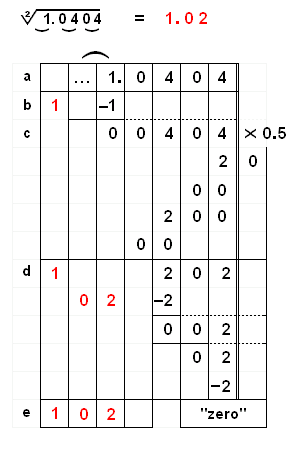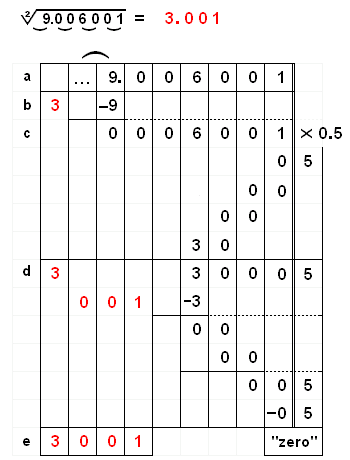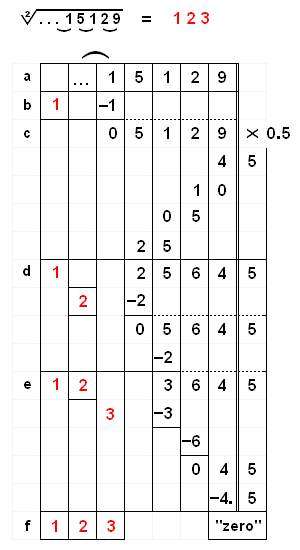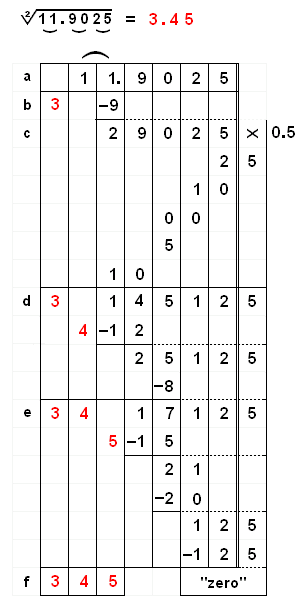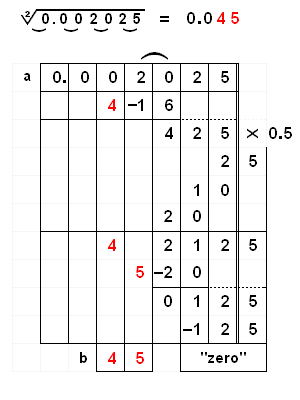PRINTER FRIENDLY PAGES

 Edvaldo Siqueira Rio de Janeiro, Brazil Totton Heffelfinger Toronto Ontario, Canada